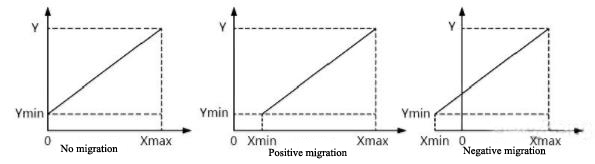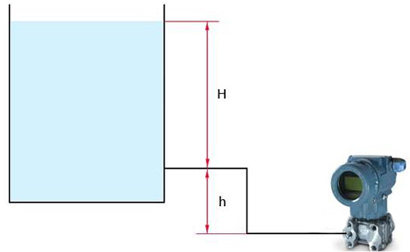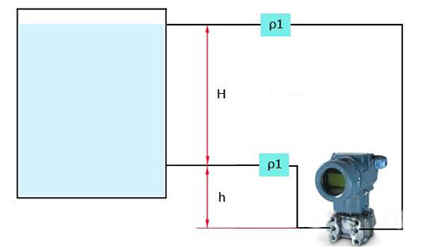Pressure Sensor

Home » Wiki » Zero Migration in Differential Pressure Sensor Application

# Zero Migration in Differential Pressure Sensor Application

For the application of differential pressure sensors, zero migration processing is generally not required if the measurement conditions are enough. However, the differential pressure sensor often takes into consideration for the maintenance and installation during the actual use. In order to facilitate the operation, there is often a horizontal difference between the pressure tapping point and the measuring instrument, or when there are some limiting factors in the measured medium, such as strong corrosiveness, excessive viscosity, etc., this will cause certain obstacles to the whole pressure measurement process, and even affect the measurement accuracy of the meter. Therefore, in order to achieve accurate measurement for the differential pressure sensor as much as possible, the differential pressure sensor zero-point migration technique is usually processed. This paper will conduct a detailed analysis and research on the zero-point migration for differential pressure sensors.
• Zero migration of differential pressure sensor
1. Zero migration concept
The zero-point migration means that in order to ensure the effectiveness of the pressure sensor basic quantity (mainly including range, measurement accuracy, etc.) during measurement, take the starting point of measurement as the core, implementing a safety method that change numerical value. The zero migration has a great similarity with the zero adjustment, whose significance is promoting the measurement signal of the pressure sensor matches the lower limit of the input signal. The difference between zero adjustment and zero migration depends on whether Xmin is 0. When Xmin is 0, it is zero adjustment, otherwise it is zero migration. If the end measurement gradually shows a positive value, it can be regarded as a positive migration, otherwise it is the second form of migration, called negative migration.2. Differential pressure sensor zero-point migration characteristics
The above figure shows the input and output characteristics of the differential pressure sensor during the zero-point migration process. It can be seen from the situation shown in figure 1 that the sensor slope does not change after the zero-point migration, only its input-output characteristics are slightly shifted in distance. In general, the effect of the change is not obvious, that is to say, the range of the pressure sensor is still in a constant state. Then, assuming that the range compression operation is performed on the basis of the zero point migration, the sensitivity and measurement accuracy of the differential pressure sensor are naturally consolidated.

3. Conformation of the migration amount for differential pressure sensor
The confirmation of the migration amount is the primary premise for the differential pressure sensor zero-point migration technology. Especially under the condition that the sensor used in different ranges, the migration quantity naturally has a difference. At the present stage, the confirmation standard for the differential pressure sensor migration is to regard the percentage of the sensor maximum range as the migration amount. For sensors whose zero positive and negative migration are positive and negative 100% of the maximum range, assuming that 0kPa-179.4kPa and 0kPa-29.3kPa are the two basic use ranges of the differential pressure sensor, if any pressure value in the range of 0kPa-179.4kPa is injected into the sensor high and low voltage inlet, 5mA sensor migration can be obtained. However, the 179.4 kPa pressure has reached the limit for the high pressure injection port of the differential pressure sensor. If the migration is adjusted from zero to 5 mA, the high voltage has become overpressure and the limit has been reached for the sensor zero-point migration technology. It can be seen that the measurement range of the differential pressure sensor during positive migration is determined by the sum of the usage range and the zero migration amount. If change it into the negative migration, the pressure port of the differential pressure sensor is injected with a negative pressure. Therefore, as long as the injected pressure is maintained in the range of 0kPa-179.4kPa, the measurement limit will always be outside the sum of the differential pressure and the zero migration amount. That is to say, there is no zero migration limit for the negative migration of the differential pressure sensor.
• Differential pressure sensor zero-point migration classification
In essence, zero migration includes three forms: no migration, positive migration and negative migration. Since no migration is rare, this paper only introduces the positive and negative migration for the differential pressure sensor.

1. Positive migration
The difference between the installation position of differential pressure sensor and the measured horizontal height of liquid level is a common phenomenon in actual measurement. The above figure shows a form of migration.According to observation, the container is an open container, and h is the horizontal height difference between the installation position of the differential pressure sensor and the measured liquid level, and further, a functional relationship ΔP=ρgH+ρgh that associated with pressure difference ΔP can be obtained. Assuming that the output pressure of the differential pressure sensor is greater than 4 mA, it must be ensured that a portion of the static pressure remains in the positive pressure chamber of the sensor. In addition, it is assumed that the installation position of the differential pressure sensor is kept at the same level as the measured liquid level, that is, H is 0. Then, when H is taken as the maximum value, the equation of ΔP=ρgH+ρgh can be obtained again. At this time, the output pressure of the sensor has exceeded the limit value of 20 mA. It is thus stated that the static pressure generated by ρgh is an extra part and must be eliminated, thereby obtaining a type of migration, which is called positive migration.

2. Negative migration
Figure 3 shows the principle of negative migration. In the operation of the pressure chamber in the differential pressure sensor, if liquid or gas in the closed container is injected into the pressure chamber, the measuring pipeline will suffer a great degree of damage and even suffer severe corrosion. In order to avoid the liquid or gas flowing in the closed container, isolating tanks are respectively installed between the positive and negative pressure chambers of the differential pressure sensor and the pressure tapping point, then inject isolation fluid with a density of ρ1.If assuming that H are 0 and the maximum value respectively, then two different numerical conditions of the pressure difference ΔP can be obtained. It also shows that when H is 0, 4 mA is the limit value of the differential pressure sensor, but when H is the maximum value, the actual density of the isolation fluid is far beyond the expected value. Therefore, when the liquid level is at the highest position, since the pressure in the negative pressure chamber is larger than the pressure in the positive pressure chamber, the actual output pressure value of the liquid level meter does not match the theoretical estimated value. It seriously affects the balance between the liquid level and the output pressure of the differential pressure sensor. Through analysis, in order to maintain the relationship between the actual liquid level and the instrument, the static pressure from the pressure line in the negative pressure chamber should be removed, which is the most fundamental method. During this period, the negative migration technique for the differential pressure sensor is required, and the migration amount can be regarded as ρ1gh.

• Discussion on the malfunction in differential pressure sensor zero migration
1. Positive migration malfunction
In the process of using the differential pressure sensor, when judging its measurement accuracy in the positive migration state, there will be two problems as the following.
1.1 Instrument output error
If the normal operation sequence is followed, the positive and negative pressure measuring chambers in three valves manifold of the differential pressure sensor should be closed first, then the balance valve opening and the instrument plug emptying should be performed. The 4mA limit should be much higher than the meter output value. If the instrument output value is greater than the limit value, it can indicates that the operating device is clogged. The most likely fault location is the three valves manifold and the lead of positive pressure chambers.
1.2 The migration amount or zero display is not normal
If the pressure point in the positive pressure chamber and the vent switch are sequentially turned off and turned on, the meter should display 4 mA if the output is normal. Then when the migration amount or the zero position value is small, the instrument output value will be significantly lower than 4mA. On the other hand, if the output value of the meter is greater than 4 mA, then the zero or the migration amount will increase. In addition, if the isolation fluid leaks or is not filled, the isolation fluid will penetrate into the positive and negative pressure chambers.

2. Negative migration malfunction
In the process of using the differential pressure sensor, when judging its measurement accuracy in the negative migration state, there will be generally two operations. First, the positive and negative pressure measuring chambers in three valves manifold of the differential pressure sensor should be closed. Then the balance valve opening and the instrument plug emptying should be performed. At this time, the instrument output value should be 20mA. Then sequentially turn off and turn on the pressure point in the positive pressure chamber and the vent switch. The instrument output value should be 4mA. After two operations, if the meter output value does not match the actual value, then the problem may appear in the following aspects:
2.1 Isolation fluid leakage.
2.2 The leads of the positive and negative pressure chambers are not circulating.
2.3 Zero is not accurate.
2.4 Migration amount has changed.
Controlling the liquid level correctly is the prerequisite for the stable operation of the production equipment. Only by mastering the principle of zero-point migration for differential pressure sensors can it be used flexibly in practical applications to timely and accurately handle faults in field instruments and improve control schemes.

I have an Opinion:

Plz Calculate (5 * 3) =
(Your comment will show after approved.)

You may also like: